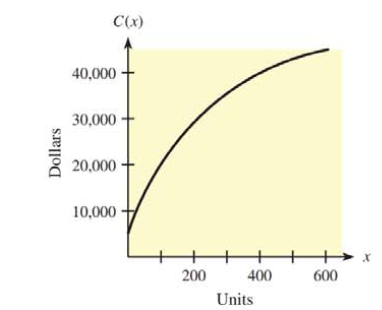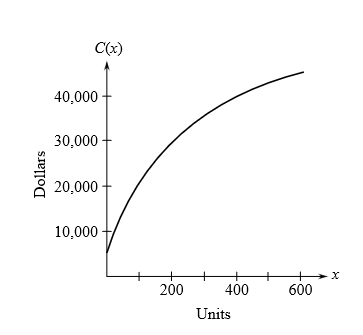Chapter 9.9, Problem 23E### Mathematical Applications for the ...

11th Edition
Ronald J. Harshbarger + 1 other
ISBN: 9781305108042

#### Solutions

Chapter
Section### Mathematical Applications for the ...

11th Edition
Ronald J. Harshbarger + 1 other
ISBN: 9781305108042
Textbook Problem

# In each of Problems 23 and 24, the graph of a company’s total cost function is shown. For each problem, use the graph to answer the following questions.(a) Will the 101st item or the 501st item cost more to produce? Explain.(b) Does this total cost function represent a manufacturing process that is getting more efficient or less efficient? Explain.(a)

To determine

Whether the 101st item or 501st item cost more to produce by the use of the graph of the total cost function,Explanation

Given Information:

The graph of the cost function,

Explanation:

Consider the provided graph,

(b)

To determine

Whether the total cost function represents the more efficient or less efficient manufacturing process by the use of the graph of total cost function,### Still sussing out bartleby?

Check out a sample textbook solution.

See a sample solution

#### The Solution to Your Study Problems

Bartleby provides explanations to thousands of textbook problems written by our experts, many with advanced degrees!

Get Started

#### Find more solutions based on key concepts